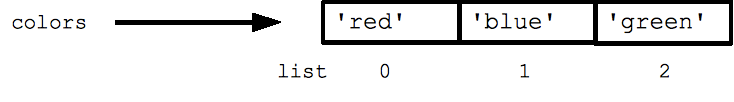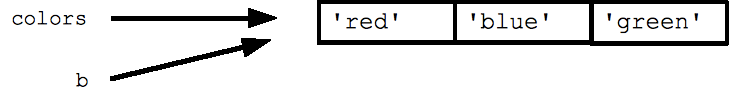# Python Lists

Python has a great built-in list type named "list". List literals are written within square brackets [ ]. Lists work similarly to strings -- use the len() function and square brackets [ ] to access data, with the first element at index 0. (See the official python.org list docs.)

```  colors = ['red', 'blue', 'green']
print(colors)    ## red
print(colors)    ## green
print(len(colors))  ## 3
```Assignment with an = on lists does not make a copy. Instead, assignment makes the two variables point to the one list in memory.

```  b = colors   ## Does not copy the list
```The "empty list" is just an empty pair of brackets [ ]. The '+' works to append two lists, so [1, 2] + [3, 4] yields [1, 2, 3, 4] (this is just like + with strings).

## FOR and IN

Python's *for* and *in* constructs are extremely useful, and the first use of them we'll see is with lists. The *for* construct -- `for var in list` -- is an easy way to look at each element in a list (or other collection). Do not add or remove from the list during iteration.

```  squares = [1, 4, 9, 16]
sum = 0
for num in squares:
sum += num
print(sum)  ## 30
```

If you know what sort of thing is in the list, use a variable name in the loop that captures that information such as "num", or "name", or "url". Since Python code does not have other syntax to remind you of types, your variable names are a key way for you to keep straight what is going on. (This is a little misleading. As you gain more exposure to python, you'll see references to type hints which allow you to add typing information to your function definitions. Python doesn't use these type hints when it runs your programs. They are used by other programs such as IDEs (integrated development environments) and static analysis tools like linters/type checkers to validate if your functions are called with compatible arguments.)

The *in* construct on its own is an easy way to test if an element appears in a list (or other collection) -- `value in collection` -- tests if the value is in the collection, returning True/False.

```  list = ['larry', 'curly', 'moe']
if 'curly' in list:
print('yay') ## yay
```

The for/in constructs are very commonly used in Python code and work on data types other than list, so you should just memorize their syntax. You may have habits from other languages where you start manually iterating over a collection, where in Python you should just use for/in.

You can also use for/in to work on a string. The string acts like a list of its chars, so `for ch in s: print(ch)` prints all the chars in a string.

### Range

The range(n) function yields the numbers 0, 1, ... n-1, and range(a, b) returns a, a+1, ... b-1 -- up to but not including the last number. The combination of the for-loop and the range() function allow you to build a traditional numeric for loop:

```  ## print the numbers from 0 through 99
for i in range(100):
print(i)
```

There is a variant xrange() which avoids the cost of building the whole list for performance sensitive cases (in Python 3, range() will have the good performance behavior and you can forget about xrange()).

### While Loop

Python also has the standard while-loop, and the *break* and *continue* statements work as in C++ and Java, altering the course of the innermost loop. The above for/in loops solves the common case of iterating over every element in a list, but the while loop gives you total control over the index numbers. Here's a while loop which accesses every 3rd element in a list:

```  ## Access every 3rd element in a list
i = 0
while i < len(a):
print(a[i])
i = i + 3
```

### List Methods

Here are some other common list methods.

• list.append(elem) -- adds a single element to the end of the list. Common error: does not return the new list, just modifies the original.
• list.insert(index, elem) -- inserts the element at the given index, shifting elements to the right.
• list.extend(list2) adds the elements in list2 to the end of the list. Using + or += on a list is similar to using extend().
• list.index(elem) -- searches for the given element from the start of the list and returns its index. Throws a ValueError if the element does not appear (use "in" to check without a ValueError).
• list.remove(elem) -- searches for the first instance of the given element and removes it (throws ValueError if not present)
• list.sort() -- sorts the list in place (does not return it). (The sorted() function shown later is preferred.)
• list.reverse() -- reverses the list in place (does not return it)
• list.pop(index) -- removes and returns the element at the given index. Returns the rightmost element if index is omitted (roughly the opposite of append()).

Notice that these are *methods* on a list object, while len() is a function that takes the list (or string or whatever) as an argument.

```  list = ['larry', 'curly', 'moe']
list.append('shemp')         ## append elem at end
list.insert(0, 'xxx')        ## insert elem at index 0
list.extend(['yyy', 'zzz'])  ## add list of elems at end
print(list)  ## ['xxx', 'larry', 'curly', 'moe', 'shemp', 'yyy', 'zzz']
print(list.index('curly'))    ## 2

list.remove('curly')         ## search and remove that element
list.pop(1)                  ## removes and returns 'larry'
print(list)  ## ['xxx', 'moe', 'shemp', 'yyy', 'zzz']
```

Common error: note that the above methods do not *return* the modified list, they just modify the original list.

```  list = [1, 2, 3]
print(list.append(4))   ## NO, does not work, append() returns None
## Correct pattern:
list.append(4)
print(list)  ## [1, 2, 3, 4]
```

### List Build Up

One common pattern is to start a list as the empty list [], then use append() or extend() to add elements to it:

```  list = []          ## Start as the empty list
list.append('a')   ## Use append() to add elements
list.append('b')
```

### List Slices

Slices work on lists just as with strings, and can also be used to change sub-parts of the list.

```  list = ['a', 'b', 'c', 'd']
print(list[1:-1])   ## ['b', 'c']
list[0:2] = 'z'    ## replace ['a', 'b'] with ['z']
print(list)         ## ['z', 'c', 'd']
```

## Exercise: list1.py

To practice the material in this section, try the problems in list1.py that do not use sorting (in the Basic Exercises).

[{ "type": "thumb-down", "id": "missingTheInformationINeed", "label":"Missing the information I need" },{ "type": "thumb-down", "id": "tooComplicatedTooManySteps", "label":"Too complicated / too many steps" },{ "type": "thumb-down", "id": "outOfDate", "label":"Out of date" },{ "type": "thumb-down", "id": "samplesCodeIssue", "label":"Samples / code issue" },{ "type": "thumb-down", "id": "otherDown", "label":"Other" }]
[{ "type": "thumb-up", "id": "easyToUnderstand", "label":"Easy to understand" },{ "type": "thumb-up", "id": "solvedMyProblem", "label":"Solved my problem" },{ "type": "thumb-up", "id": "otherUp", "label":"Other" }]